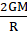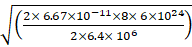### Solve the following problems. If mass of a planet is eight times the mass of the earth and its radius is twice the radius of the earth, what will be the escape velocity for that planet?

Question 9.

Solve the following problems.

If mass of a planet is eight times the mass of the earth and its radius is twice the radius of the earth, what will be the escape velocity for that planet?

Answer:

Mass of planet M = 8 x mass of earth = 8 x 6 x 1024 kg

Radius of planet R = 2 x radius of earth = 2 x 6.4 x 106 m

G = gravitational constant = 6.67 x 10-11 N m2/kg2

So escape velocity = √Escape velocity == 22.4 km/sec.

So escape velocity of the planet will be 22.4 km/sec.